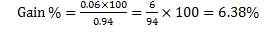# IAS Exam:IAS Prelims CSAT Basic Numeracy Set 5

Here, we have provided the questions of Basic Numeracy for the Civil Services CSAT Paper II of UPSC IAS Exam 2016. The pattern of these Basic Numeracy Multiple Choice Questions has been kept in line with the questions asked in earlier attempts of Civil Services IAS Exam.

Created On: Mar 29, 2016 09:24 IST
Modified On: Mar 29, 2016 11:37 ISTIn the Civil Services Prelim Examination, the CSAT paper has been made qualifying in nature from 2015 onwards. The candidates have to score at least qualifying marks in the paper but in case, if a candidate could not be able to score the required qualifying marks in CSAT Paper then his/her other papers will not be evaluated. So, it is important for the candidates to do preparation in such a manner that there must not lay them in any suspicion of underscoring.

3rd set of Basic Numeracy for CSAT Exam 2016 (20 questions) – 22nd March 2016

Category: Ratio and proportion

1. In what ratio should the 2 mixtures of milk containing milk and water in ratios 3 : 4 and 10 : 7 should be mixed so that the resulting mixture has equal amount of milk and water?

(a) 21 : 17
(b) 13 : 11
(c)   9 : 5
(d) 17 : 13

Explanation:
By Alligation,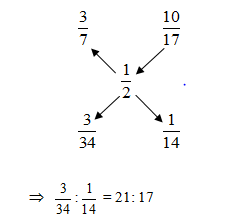Category: Averages

2.Number of members in the upper house and the lower house of parliament is 200 and 300 respectively. The average age of the members of Lower house and Upper house is 40 years and 50 years respectively. A member of the Upper house leaves and becomes the member of the Lower house. Thus, the average age of both the houses increases. Which one of the following statements is true?

(a) The age of this member is greater than 50 years.
(b) The age of this member is less than 40 years
(c) The age of this member is greater than 40 but less than 50 years
(d) The age of the member is 50 years

Explanation: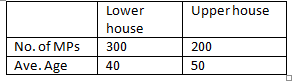Since when a member of Upper house joins the Lower house and the average age of both the houses increases, it means the average age of this member must lie between 40 and 50. When the age of this member is greater than 40, then the average age of the Lower house will increase. Again when the age of this member is less than 50, then after leaving it, the average age of the Upper house will increase.

3.In an NGO, the daily average wages of 20 illiterate employees is decreased from Rs. 25 to Rs. 10, thus the average salary of all the literate (educated) and illiterate employees is decreased by Rs. 10 per day. The number of educated employees working in the NGO is:

(a) 15
(b) 20
(c) 10
(d) 25

Explanation:
Let the number of literate employees be l and the average salary of literate employees be s.
Then,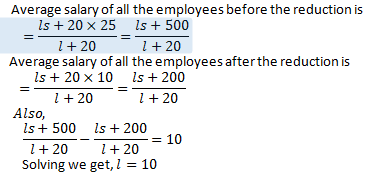Category: Speed and distance

4.In special race, one racer leaves from A to reach B at 6:00 am. After 2 hours other racer leaves from B to reach A. If first racer reaches B at 10:00am while the second racer reaches A at 11:30 am, at what time they will cross each other?
(a) 8:00am
(b) 7:28am
(c) 8:56am

Explanation:
Let the distance between A and B be ‘x’ km and the time after which they cross each other be ‘y’ hours.

Clearly,     First racer covers x km in: (10 – 6) = 4 hours
Second racer covers x km in: (11:30 – 8) = 7/2 hours

Thus,   Speed of first racer =x/4  kmph

Speed of second racer =2x/7  kmph

Now,    Distance covered by first racer in (y+2) hrs + Distance covered in y hrs = x

x/4 (y+2)+2x/7  ×y=x
(y+2)/4+y/7=1
7y+14+11y=28
y=14/15  hrs
y=14/15×60=56 mins

Therefore, the racer will meet at 8:00+ 56mins = 8:56 am

Category: Permutation and combination

5.From a group of 6 all-rounder and 5 bowlers a bowling attack of 5 has to be picked, such that it contains atleast 2 bowlers. In how many ways can this be done?

(a) 462
(b) 381
(c) 278
(d) None of these

Explanation:
The number of ways is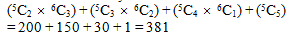6.If all the words which can be written using the alphabets of the word TABLE are written in an order then what will be the position of TABLE?

(a) 50
(b) 96
(c) 97
(d) 98

Explanation:
Words starting with A = 24
Words starting with B = 24
Words starting with E = 24
Words starting with L = 24

Total = 96
Now 97th word is TABEL
98th word = TABLE

Category: Number system

7. If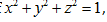, then which of the following cannot be a value of (xy + yz + zx)?

(a) 0
(b) 1/2
(c)-1/4
(d)-1

Explanation: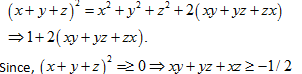Option (d) is not in this range and is hence not possible

Category: Time and Work

8.When working together, A and B can finish a task in 30 days. A and B start the task but after 16 days, A leaves. B then finishes the remaining work alone in 44 days. If B was supposed to finish the work alone, how long will it take?

(a) 30 days
(b) 40 days
(c) 60 days
(d) 70 days

Explanation:

Let their respective efficiencies be A & B
In 16 days, work done by A = 16A
In 44 days, work done by B = 44B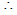16A + 44B = 1 ………. (1)
[Efficiency of complete work is unity]
Also,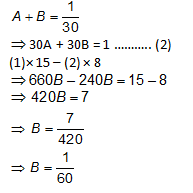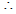Time taken by B = 60 days.

9. An inlet pipe fills water in a reservoir at the rates of 0.1 litres/sec. When the reservoir is full, the inlet is opened but due to a leak at the bottom, it is emptied in 12 hours. What is the capacity of the reservoir, if it is known that the leak can empty the full reservoir in 8 hours had there been no inlet?

(a)7500
(b) 7690
(c) 8400
(d) 8640

Explanation:

Let the capacity to reservoir be ‘x’ litres
Then inlet pipe can fill it in x/0.1 seconds =10x seconds =  x/360 hours
Now, leak can empty in 8 hrs
When both inlet and leak are working, time required to empty the full reservoir =1/8–360/x
Also,
1/8-360/x=1/12
Solving,x=8640

Category: Profit and Loss

10. Find the gain percent if a dishonest shopkeeper sells the goods at cost price but reduces the weight to 940 gm for the 1 kg. ?
(a) 6.34%
(b) 6.38%
(c) 6.35%
(d) 6.39%

Explanation:

Let the cost of goods be Rs.1 for the kg.

Then he sell his good for Rs.1 which cost him Rs. 0.94, Gain on Rs.0.94 = 0.06